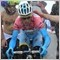# Everything about RSI - page 65216389

This is a result of an tradestation experiment. It is a rsi of a rsi of a ma (sort of a double rsi of a moving average)

Files:1232

mladen:
This is a result of an tradestation experiment. It is a rsi of a rsi of a ma (sort of a double rsi of a moving average)

Thank you216389

3 time frame Connors RSI posted here : https://www.mql5.com/en/forum/174385/page1392047

Rsi with step ma oscillator (by Alexander Gettinger)

Files:216389

RSI of MACD double

This is a rsi of macd double (it was posted here : https://www.mql5.com/en/forum/178018/page60 ). As it was already told, rsi can be used as a kind of normalization method for macd (since macd is un-bound indicator) and to enhance macd.

Upper is the regular macd double lower is the resi of that same macd double. Signals on rsi of macd double tend to be a bar or two faster than on the macd double itself (it is due to the nature of rsi)

Files:23

Thanks for the indi share!676

Look nice. Will test it346

mrtools:
Drunkpips, just guessing you meant crossing 50, that's how i made this one.

please can you add momentum 25 to this indicator for me pls

also , i will appreciate if you can also add rsi 25 to the CCI-volume weighted indicator coded by mladen

thanks216389

drunkpips:
please can you add momentum 25 to this indicator for me pls

also , i will appreciate if you can also add rsi 25 to the CCI-volume weighted indicator coded by mladen

thanks

drunkpips

Momentum and RSI are indicators with entirelly different set of values produced. If you place momentum in that same subwindow, momentum would look like a flat line. Same thing goes for CCI and SI : different values altogether. In the case of CCI RSI combination rsi would be "flatened"216389

Here is what would a rsi + momentum combination look like. Attaching the template that made that too. Green line is momentum, blue line is rsi

Files: